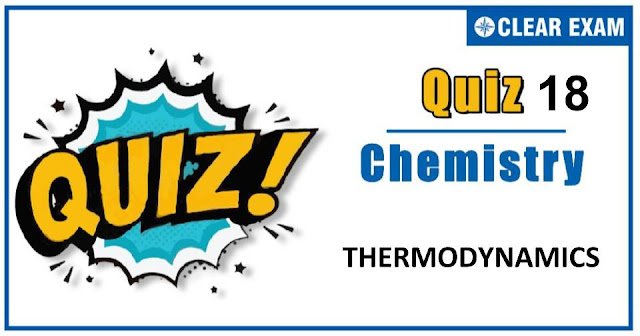## Thermodynamics Quiz-18

Thermodynamics is a very important branch of both chemistry and physics. It deals with the study of energy, the conversion of energy between different forms and the ability of energy to do work. Thermodynamics is an important chapter for JEE Mains, JEE Advance NEET and many others exams. As this chapter is present in both chemistry and physics and there is only a minor difference between them hence it becomes more important topic. It is not a tough topic, it only requires practice. This topic has been given good weightage in all engineering exams. So don't skip this and prepare well. All the best !.

Q1. The enthalpy of combustion of H2, cyclohexane(C6H10) and cyclohexane(C6H12) are −241, −3800 and −3920 kJ per mol respectively. Heat of hydrogenation of cyclohexane is
•  +121 kJ/mol
•  −121 kJ/mol
•  +242 kJ/mol
•  −242 kJ/mol
Solution:-

Q2. Δn values in ΔH = ΔU + ΔnRT may have:
•  Integer nature
•  Fractional value
•  Positive or negative
•  All of these
Solution:-
Δn depends on stoichiometry of reaction.

Q3. Given enthalpy of formation of CO2(g) and CaO(s) are −94.0 kJ and −152 kJ respectively and the enthalpy of the reaction, CaCO3(s) → CaO(s) + CO2(g) is 42 kJ. The enthalpy of formation of CaCO3(s) is :
•  −42 kJ
•  −202 kJ
•  +202 kJ
•  −288 kJ
Solution:-

Q4. The process in which pressure remains constant throughout a change is:
•  Isochoric
•  Isobaric
•  Isothermal
Solution:-
An isobaric process is one in which changes are made at constant pressure.

Q5. Which is correct about the heat of combustion?
•  The combustion be exothermic in some cases and endothermic in other cases
•  Heat of combustion is always exothermic
•  Its value change with temperature
•  All of the above
Solution:-
Heat of combustion is always exothermic; Few combustion reactions such as F2 to F2O, N2 to N2O and NO are endothermic but these reactions do not give heat of combustion because the substance should be completely oxidized. In F2O, F2 is reduced and N2O and NO are not completely oxidized state of N2. However, three reactions are exceptions but these do not represent heat of combustion. These are,
N2 + O2 → N2O;   ΔH = +ve
N2 + O2 → NO;   ΔH = +ve
and F2 + ½O2 → F2O;   ΔH = +ve

Q6. The internal energy change when a system goes from state A to B is 40 kJ/mol. If the system goes from A to B by a reversible path and returns to state A by an irreversible path, what would be the net change in internal energy?
•  40 KJ
•  > 40 kJ
•  < 40 kJ
•  Zero
Solution:-
In a cyclic process, ΔE = 0

Q7. For an adiabatic expansion of a perfect gas ΔP/P is equal to:
•  ΔVV
•  γΔVV
•  γΔVV
•  γ2ΔVV
Solution:-

Q8. Which one of the following is an exothermic reaction?
•  N2(g) + O2(g) + 180.8 kJ → 2NO(g)
•  N2(g) + 3H2(g) − 92 kJ → 2NH3(g)
•  C(g) + H2O → CO(g) + H2(g) − 131.1 kJ
•  Cgraphite + 2S(s) → CS2(l) − 91.9 kJ
Solution:-
The reactions in which products has lesser energy than reactants, then energy is released in the reaction and such reactions are known as exothermic reactions e.g.,
N2(g) + 3H2(g) → 2NH3(g) + 92 kJ
In this equation energy is released, so, it is an example of exothermic reaction.

Q9. For the reaction, C (graphic) + ½O2(g) → CO(g) at 298 K and 1 atm, ΔH = −26.4 kcal. What is ΔE, if the molar volume of graphite is 0.0053 L? ( R = 0.002 kcal mol−1 K−1 )
•  −26.7 kcal
•  +26.7 kcal
•  −52.4 kcal
•  +52.4 kcal
Solution:-

Q10. If 50 calorie are added to a system and system does work of 30 calorie on surroundings, the change in internal energy of system is:
•  20 cal
•  50 cal
•  40 cal
•  30 cal
Solution:-
q = ΔU + W
50 = ΔU + 30
∴ ΔU = 20 cal## Want to know more

Please fill in the details below:

## Latest NEET Articles\$type=three\$c=3\$author=hide\$comment=hide\$rm=hide\$date=hide\$snippet=hide

Name

ltr
item
BEST NEET COACHING CENTER | BEST IIT JEE COACHING INSTITUTE | BEST NEET & IIT JEE COACHING: Thermodynamics Quiz-18
Thermodynamics Quiz-18
https://1.bp.blogspot.com/-ofS-kRv0_YA/X5l2kUn_D3I/AAAAAAAAB5A/ln1VmGOMT7Y3nk-mytwZw3jvuCaLVYxUACLcBGAsYHQ/w640-h336/Quiz%2BImage%2B20%2B%252814%2529.jpg
https://1.bp.blogspot.com/-ofS-kRv0_YA/X5l2kUn_D3I/AAAAAAAAB5A/ln1VmGOMT7Y3nk-mytwZw3jvuCaLVYxUACLcBGAsYHQ/s72-w640-c-h336/Quiz%2BImage%2B20%2B%252814%2529.jpg
BEST NEET COACHING CENTER | BEST IIT JEE COACHING INSTITUTE | BEST NEET & IIT JEE COACHING
https://www.cleariitmedical.com/2020/10/thermodynamics-quiz-18.html
https://www.cleariitmedical.com/
https://www.cleariitmedical.com/
https://www.cleariitmedical.com/2020/10/thermodynamics-quiz-18.html
true
7783647550433378923
UTF-8

STAY CONNECTED